Next: Two-Dimensional Fourier Optics Up: Wave Optics Previous: Single-Slit Diffraction

# Multi-Slit Diffraction

Suppose that the opaque screen in our interference/diffraction apparatus contains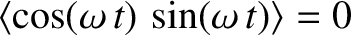identical, equally spaced, parallel slits of finite width. Let the slit spacing be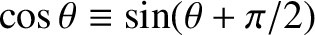, and the slit width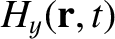, where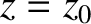. It follows that the aperture function for the screen is written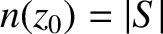(1051)

where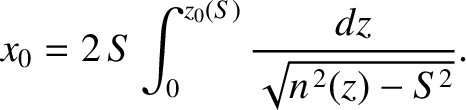(1052)

and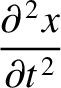(1053)

We recognize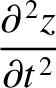as the aperture function for a single slit, of finite width, that is centered on. [See Equation (1046).]

Assuming normal incidence (i.e.,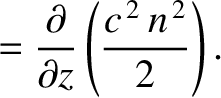), the interference/diffraction function, which is the Fourier transform of the aperture function, takes the form [see Equation (1044)]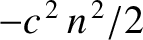(1054)

Hence,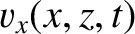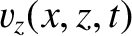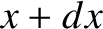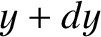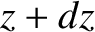(1055)

where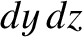. Here, we have made use of the result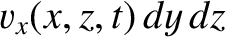, for any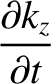, which follows because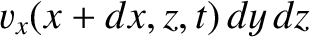is even in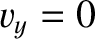, whereas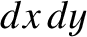is odd. We have also employed the trigonometric identity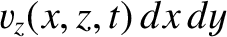. (See Appendix B.) The previous expression reduces to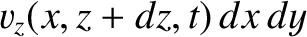(1056)

Here [cf., Equation (1030)],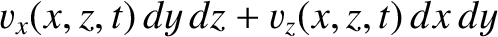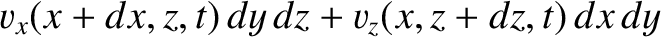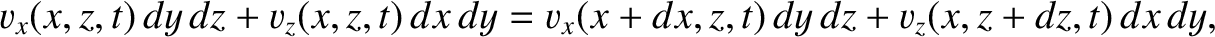(1057)

is the interference/diffraction function foridentical parallel slits of negligible width that are equally spaced a distanceapart, and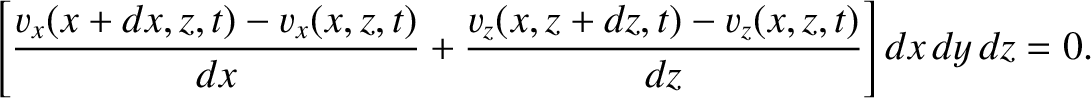(1058)

is the corresponding aperture function. Furthermore [cf., Equation (1047)],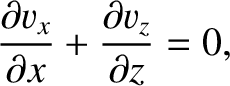(1059)

is the interference/diffraction function for a single slit of width.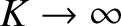We conclude, from the preceding analysis, that the interference/diffraction function foridentical, equally spaced, parallel slits of finite width is the product of the interference/diffraction function foridentical, equally spaced, parallel slits of negligible width,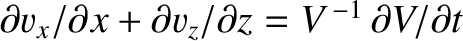, and the interference/diffraction function for a single slit of finite width,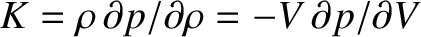. We have already encountered both of these functions. The former function (see Figure 69, which shows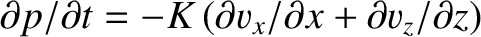) consists of a series of sharp maxima of equal amplitude located at [see Equation (1031)]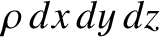(1060)

where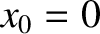is an integer. The latter function (see Figure 71, which shows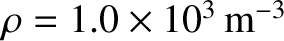) is of order unity for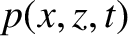, and much less than unity for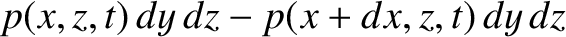. It follows that the interference/diffraction pattern associated withidentical, equally spaced, parallel slits of finite width, which is given by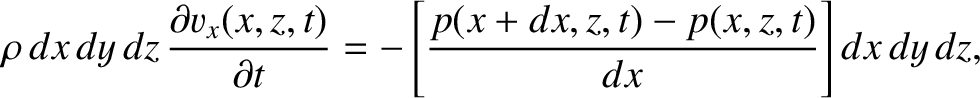(1061)

is similar to that foridentical, equally spaced, parallel slits of negligible width,, except that the intensities of the various maxima in the pattern are modulated by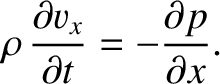. Hence, those maxima lying in the angular range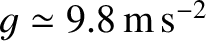are of similar intensity, whereas those lying in the range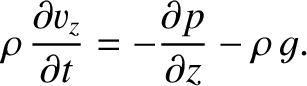are of negligible intensity. This is illustrated in Figure 73, which shows the multi-slit interference/diffraction pattern calculated for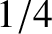,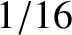, and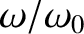. As expected, the maxima lying in the angular range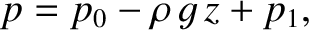have relatively large intensities, whereas those lying in the range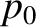have negligibly small intensities.Next: Two-Dimensional Fourier Optics Up: Wave Optics Previous: Single-Slit Diffraction
Richard Fitzpatrick 2013-04-08